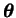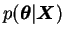Next: Laplace approximation Up: Posterior approximations Previous: Model selection   Contents

## Stochastic approximations

The basic idea in stochastic approximations is to somehow get samples from the true posterior distribution. This is usually done by constructing a Markov chain for the model parameterswhose stationary distribution corresponds to the posterior distribution. Simulation algorithms doing this are called Markov chain Monte Carlo (MCMC) methods.

The most important of such algorithms is the Metropolis-Hastings algorithm. To use it, one must be able to compute the unnormalised posterior of Equation (3.3). In addition one must specify a jumping distribution for the parameters. This can be almost any reasonable distribution that models possible transitions between different parameter values. The algorithm works by getting random samples from the jumping distribution and then either taking the suggested transition or rejecting it, depending on the ratio of the posterior probabilities of the two values in question. The normalising factor of the posterior is not needed as only the ratio of the posterior probabilities is needed .

Neal  discusses the use of MCMC methods for neural networks in detail. He also presents some modifications to the basic algorithms to improve their performance for large neural network models.Next: Laplace approximation Up: Posterior approximations Previous: Model selection   Contents
Antti Honkela 2001-05-30